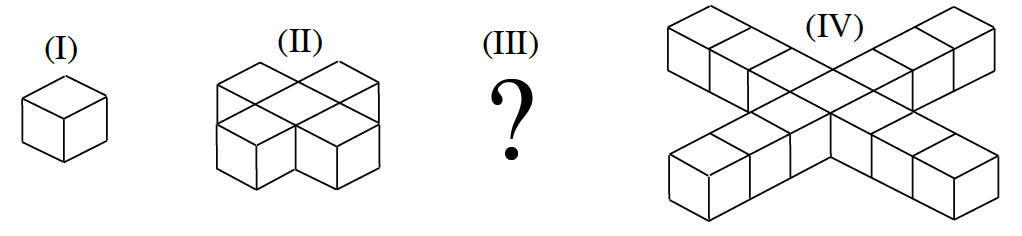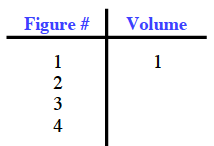### Home > CCA2 > Chapter 6 > Lesson 6.1.1 > Problem6-10

6-10.

Each cube below is 1 cm on a side.1. Based on the pattern, find the volume of Figure III.

What is the difference between Figure (II) and Figure (IV)?
Make a table.2. If the pattern continues, write an expression to represent the volume of figure N. What kind of sequence is this?

Find the common difference between the volumes of each figure.

$4n−3$, arithmetic